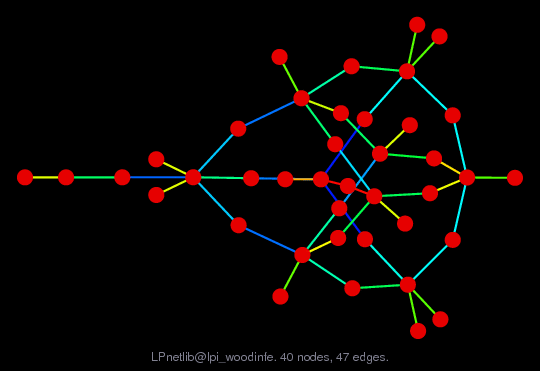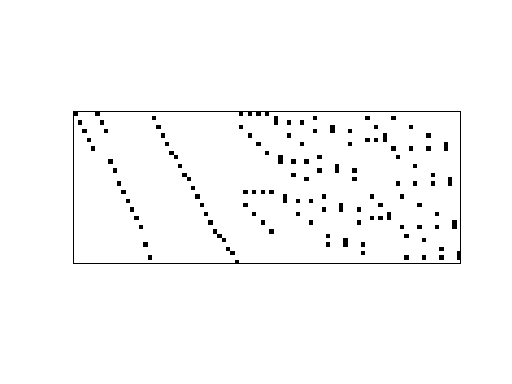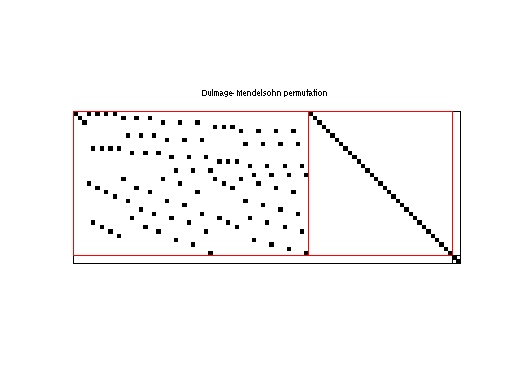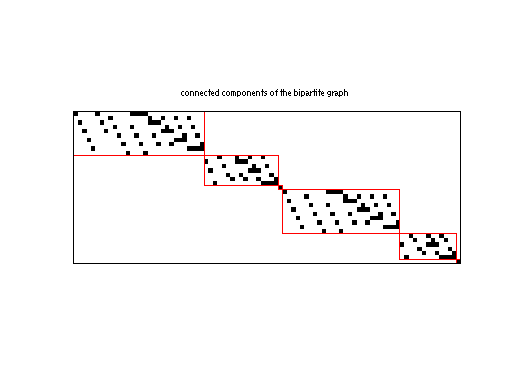Matrix: LPnetlib/lpi_woodinfe

Description: Netlib LP problem woodinfe: minimize c'*x, where Ax=b, lo<=x<=hi(bipartite graph drawing)• Matrix group: LPnetlib
• download as a MATLAB mat-file, file size: 2 KB. Use UFget(731) or UFget('LPnetlib/lpi_woodinfe') in MATLAB.

 Matrix properties number of rows 35 number of columns 89 nonzeros 140 structural full rank? yes structural rank 35 # of blocks from dmperm 3 # strongly connected comp. 6 explicit zero entries 0 nonzero pattern symmetry 0% numeric value symmetry 0% type integer structure rectangular Cholesky candidate? no positive definite? no

 author H. Greenberg editor J. Chinneck date 1989 kind linear programming problem 2D/3D problem? no

 Additional fields size and type b full 35-by-1 c full 89-by-1 lo full 89-by-1 hi full 89-by-1 z0 full 1-by-1

Notes:

```An infeasible Netlib LP problem, in lp/infeas.  For more information
send email to netlib@ornl.gov with the message:

send index from lp

The lp/infeas directory contains infeasible linear programming test problems
collected by John W. Chinneck, Carleton Univ, Ontario Canada.  The following
are relevant excerpts from lp/infeas/readme (by John W. Chinneck):

In the following, IIS stands for Irreducible Infeasible Subsystem, a set
of constraints which is itself infeasible, but becomes feasible when any
one member is removed.  Isolating an IIS from within the larger set of
constraints defining the model is one analysis approach.

PROBLEM DESCRIPTION
-------------------

FOREST6, WOODINFE:  very small problems derived from network-based
forestry models.  The IIS in FOREST6 includes most of the rows.
WOODINFE is the example problem discussed in detail in Greenberg ,
and has a very small IIS.  Contributor:  H.J.  Greenberg, University of

Name       Rows   Cols   Nonzeros Bounds      Notes
woodinfe     36     89      209   B

REFERENCES
----------

H.J.  Greenberg (1993).  "A Computer-Assisted Analysis System for
Mathematical Programming Models and Solutions:  A User's Guide for

```

 Ordering statistics: result nnz(V) for QR, upper bound nnz(L) for LU, with COLAMD 284 nnz(R) for QR, upper bound nnz(U) for LU, with COLAMD 102

 SVD-based statistics: norm(A) 2.94712 min(svd(A)) 1 cond(A) 2.94712 rank(A) 35 sprank(A)-rank(A) 0 null space dimension 0 full numerical rank? yes

 singular values (MAT file): click here SVD method used: s = svd (full (R)) ; where [~,R,E] = spqr (A') with droptol of zero status: ok